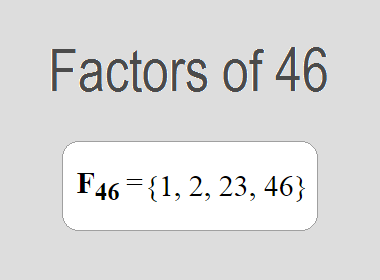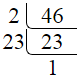# Factors of 46The factors of 46 are 1, 2, 23, and 46 i.e. F46 = {1, 2, 23, 46}. The factors of 46 are all the numbers that can divide 46 without leaving a remainder.

We can check if these numbers are factors of 46 by dividing 46 by each of them. If the result is a whole number, then the number is a factor of 46. Let's do this for each of the numbers listed above:

·        1 is a factor of 46 because 46 divided by 1 is 46.

·        2 is a factor of 46 because 46 divided by 2 is 23.

·        23 is a factor of 46 because 46 divided by 23 is 2.

·        46 is a factor of 46 because 46 divided by 46 is 1.

## How to Find Factors of 46?

1 and the number itself are the factors of every number. So, 1 and 46 are two factors of 46. To find the other factors of 46, we can start by dividing 46 by the numbers between 1 and 46. If we divide 46 by 2, we get a remainder of 0. Therefore, 2 is a factor of 46. If we divide 46 by 3, we get a remainder of 1. Therefore, 3 is not a factor of 46.

Next, we can check if 4 is a factor of 46. If we divide 46 by 4, we get a remainder of 2. Therefore, 4 is not a factor of 46. We can continue this process for all the possible factors of 46.

Through this process, we can find that the factors of 46 are 1, 2, 23, and 46. These are the only numbers that can divide 46 without leaving a remainder.

********************

********************

## Properties of the Factors of 46

The factors of 46 have some interesting properties. One of the properties is that the sum of the factors of 46 is equal to 72. We can see this by adding all the factors of 46 together:

1 + 2 + 23 + 46 = 72

Another property of the factors of 46 is that the prime factors of 46 are 2 and 23.

## Applications of the Factors of 46

The factors of 46 have several applications in mathematics. One of the applications is in finding the highest common factor (HCF) of two or more numbers. The HCF is the largest factor that two or more numbers have in common. For example, to find the HCF of 46 and 23, we need to find the factors of both numbers and identify the largest factor they have in common. The factors of 46 are 1, 2, 23, and 46. The factors of 23 are 1, and 23. The largest factor that they have in common is 23. Therefore, the HCF of 46 and 23 is 23.

Another application of the factors of 46 is in prime factorization. Prime factorization is the process of expressing a number as the product of its prime factors. The prime factors of 46 are 2 and 23, since these are the only prime numbers that can divide 46 without leaving a remainder. Therefore, we can express 46 as:

46 = 2 × 23

We can do prime factorization by division and factor tree method also. Here is the prime factorization of 46 by division method,46 = 2 × 23

Here is the prime factorization of 46 by the factor tree method,46 = 2 × 23

## Conclusion

The factors of 46 are the numbers that can divide 46 without leaving a remainder. The factors of 46 are 1, 2, 23, and 46. The factors of 46 have some interesting properties, such as having a sum of 72. The factors of 46 have several applications in mathematics, such as finding the highest common factor and prime factorization.